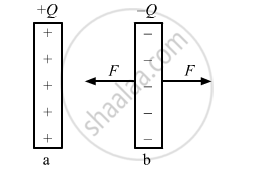# Obtain the Expression for the Energy Stored per Unit Volume in a Charged Parallel Plate Capacitor. - Physics

Obtain the expression for the energy stored per unit volume in a charged parallel plate capacitor.

#### Solution

Let us consider a parallel-plate capacitor of plate area ASuppose the plates of the capacitor are almost touching each other and a charge Q is given to the capacitor. One of the plates, say a, is kept fixed and other say b, is slowly pulled away from plate a to increase the separation from zero to d. The attractive force on the plate b at any instant due to first plate is given by:

F=Q^2/(2Aepsilon_0)

The person pulling the plate b must apply an equal and opposite force (F) in the opposite direction if the plate is moved slowly.

Work done by the person during the displacement of the second plate:

W = Fd

=(Q^2d)/(2Aepsilon_0)=Q^2/(2C)

Here,C=(Aepsilon_0)/d, which is the capacitance of the capacitor in the final position.

The work done by the person must be equal to the increase in the energy of the system.

Thus, the capacitor has a stored energy.

U=Q^2/(2C)

Now, if we pull the plates of the capacitor apart, we have to do work against the electrostatic attraction between the plates. When we increase the separation between the plates from d1 to d2, an amount Q^2/(2Aepsilon_0)(d_2-d_1) of work is performed by us and this much energy goes into the capacitor. On the other hand, new electric field is created in a volume A(d2 - d1).

The energy stored per unit volume is thus given by:

u=((Q^2(d_2-d_1))/(2Aepsilon_0))/(A(d_2-d_1))=Q^2/(2A^2epsilon_0)

1/2epsilon_0(Q/(Aepsilon_0))^2=1/2epsilon_0E^2

Here, E is the intensity of the electric field.

Concept: Energy Stored in a Capacitor
Is there an error in this question or solution?
2013-2014 (March) Delhi Set 1

Share